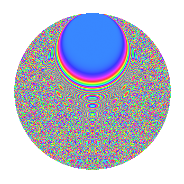# Properties

 Label 3234.2.boLevel 3234 Weight 2 Character orbit bo Rep. character $$\chi_{3234}(509,\cdot)$$ Character field $$\Q(\zeta_{30})$$ Dimension 1280 Sturm bound 1344

# Related objects

## Defining parameters

 Level: $$N$$ $$=$$ $$3234 = 2 \cdot 3 \cdot 7^{2} \cdot 11$$ Weight: $$k$$ $$=$$ $$2$$ Character orbit: $$[\chi]$$ $$=$$ 3234.bo (of order $$30$$ and degree $$8$$) Character conductor: $$\operatorname{cond}(\chi)$$ $$=$$ $$231$$ Character field: $$\Q(\zeta_{30})$$ Sturm bound: $$1344$$

## Dimensions

The following table gives the dimensions of various subspaces of $$M_{2}(3234, [\chi])$$.

Total New Old
Modular forms 5632 1280 4352
Cusp forms 5120 1280 3840
Eisenstein series 512 0 512

## Trace form

 $$1280q - 160q^{4} + 24q^{9} + O(q^{10})$$ $$1280q - 160q^{4} + 24q^{9} - 12q^{10} - 48q^{15} + 160q^{16} + 8q^{18} + 20q^{22} + 132q^{25} - 18q^{31} + 132q^{33} - 32q^{36} - 12q^{37} + 20q^{39} + 18q^{40} - 64q^{43} + 48q^{45} - 16q^{46} + 4q^{51} + 88q^{57} - 46q^{58} + 16q^{60} + 320q^{64} + 120q^{66} + 32q^{67} + 12q^{72} + 96q^{73} + 204q^{75} + 96q^{78} + 52q^{79} + 68q^{81} - 24q^{82} - 368q^{85} + 228q^{87} + 10q^{88} - 22q^{93} + 144q^{94} - 48q^{99} + O(q^{100})$$

## Decomposition of $$S_{2}^{\mathrm{new}}(3234, [\chi])$$ into newform subspaces

The newforms in this space have not yet been added to the LMFDB.

## Decomposition of $$S_{2}^{\mathrm{old}}(3234, [\chi])$$ into lower level spaces

$$S_{2}^{\mathrm{old}}(3234, [\chi]) \cong$$ $$S_{2}^{\mathrm{new}}(231, [\chi])$$$$^{\oplus 4}$$$$\oplus$$$$S_{2}^{\mathrm{new}}(462, [\chi])$$$$^{\oplus 2}$$$$\oplus$$$$S_{2}^{\mathrm{new}}(1617, [\chi])$$$$^{\oplus 2}$$

## Hecke characteristic polynomials

There are no characteristic polynomials of Hecke operators in the database